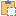#### Transform Property

The transform from the coordinate space of the box to the model coordinate space.

Namespace: Autodesk.Revit.DB
Assembly: RevitAPI (in RevitAPI.dll) Version: 17.0.0.0 (17.0.1090.0)

# Syntax

C#
``public Transform Transform { get; set; }``
Visual Basic
``````Public Property Transform As Transform
Get
Set``````
Visual C++
``````public:
property Transform^ Transform {
Transform^ get ();
void set (Transform^ value);
}``````

# Remarks

The transform must always be right-handed and orthonormal.

# ExamplesCopy C#
``````private void RotateBoundingBox(View3D view3d)
{
if (!view3d.IsSectionBoxActive)
{
TaskDialog.Show("Revit","The section box for View3D isn't active.");
return;
}
BoundingBoxXYZ box = view3d.GetSectionBox();

// Create a rotation transform to apply to the section box
XYZ origin = new XYZ(0, 0, 0);
XYZ axis = new XYZ(0, 0, 1);

// Rotate 30 degrees
Transform rotate = Transform.CreateRotationAtPoint(axis, Math.PI/6.0, origin);

// Transform the View3D's section box with the rotation transform
box.Transform = box.Transform.Multiply(rotate);

// Set the section box back to the view (requires an open transaction)
view3d.SetSectionBox(box);
}``````Copy VB.NET
``````Private Sub RotateBoundingBox(view3d As View3D)
If Not view3d.IsSectionBoxActive Then
TaskDialog.Show("Revit", "The section box for View3D isn't active.")
Return
End If
Dim box As BoundingBoxXYZ = view3d.GetSectionBox()

' Create a rotation transform to apply to the section box
Dim origin As New XYZ(0, 0, 0)
Dim axis As New XYZ(0, 0, 1)

' Rotate 30 degrees
Dim rotate As Transform = Transform.CreateRotationAtPoint(axis, Math.PI / 6.0, origin)

' Transform the View3D's section box with the rotation transform
box.Transform = box.Transform.Multiply(rotate)

' Set the section box back to the view (requires an open transaction)
view3d.SetSectionBox(box)
End Sub``````